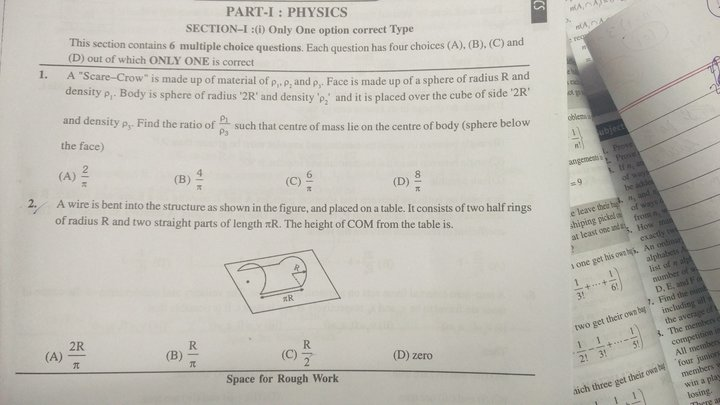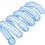# Solutions to Some Random QuestionsQuestion 1:

The height of the center of mass is a mass-weighted sum of the coordinates of the individual centers of mass.

$y_{CM} = \frac{y_1 M_1 + y_2 M_2 + y_3 M_3}{M_1 + M_2 + M_3}$

Values of these quantities:

$y_1 = 7R \hspace{1cm} M_1 = \frac{4}{3} \pi R^3 \rho_1 \\ y_2 = 4R \hspace{1cm} M_2 = \frac{32}{3} \pi R^3 \rho_2 \\ y_3 = R \hspace{1cm} M_3 = 8 R^3 \rho_3$

Plugging in:

$y_{CM} = \frac{7R \times \frac{4}{3} \pi R^3 \rho_1 + 4R \times \frac{32}{3} \pi R^3 \rho_2 + R \times 8 R^3 \rho_3}{\frac{4}{3} \pi R^3 \rho_1 + \frac{32}{3} \pi R^3 \rho_2 + 8 R^3 \rho_3 } = 4R \\ \frac{28}{3} \pi R^4 \rho_1 + \frac{128}{3} \pi R^4 \rho_2 + 8 R^4 \rho_3 = \frac{16}{3} \pi R^4 \rho_1 + \frac{128}{3} \pi R^4 \rho_2 + 32 R^4 \rho_3 \\ \frac{28}{3} \pi R^4 \rho_1 + 8 R^4 \rho_3 = \frac{16}{3} \pi R^4 \rho_1 + 32 R^4 \rho_3 \\ 4 \pi R^4 \rho_1 = 24 R^4 \rho_3 \\ \frac{\rho_1}{\rho_3} = \boxed{\frac{6}{\pi}}$

Question 2:

The height of the center of mass is a length-weighted sum of the coordinates of the individual centers of mass of the straight and curved parts. We can use length instead of mass because of the uniform mass density per unit length.

$y_{CM} = \frac{y_1 L_1 + y_2 L_2 }{L_1 + L_2 }$

Straight Part:

$L_1 = \pi R \hspace{1cm} y_1 = 0$

Curved Part:

$L_2 = \pi R \hspace{1cm} y_2 = \frac{2R}{\pi}$

Plugging in:

$y_{CM} = \frac{0 \pi R + \frac{2R}{\pi} \pi R }{\pi R + \pi R} = \boxed{\frac{R}{\pi}}$Note by Steven Chase
3 years, 8 months ago

This discussion board is a place to discuss our Daily Challenges and the math and science related to those challenges. Explanations are more than just a solution — they should explain the steps and thinking strategies that you used to obtain the solution. Comments should further the discussion of math and science.

When posting on Brilliant:

• Use the emojis to react to an explanation, whether you're congratulating a job well done , or just really confused .
• Ask specific questions about the challenge or the steps in somebody's explanation. Well-posed questions can add a lot to the discussion, but posting "I don't understand!" doesn't help anyone.
• Try to contribute something new to the discussion, whether it is an extension, generalization or other idea related to the challenge.
• Stay on topic — we're all here to learn more about math and science, not to hear about your favorite get-rich-quick scheme or current world events.

MarkdownAppears as
*italics* or _italics_ italics
**bold** or __bold__ bold
- bulleted- list
• bulleted
• list
1. numbered2. list
1. numbered
2. list
Note: you must add a full line of space before and after lists for them to show up correctly
paragraph 1paragraph 2

paragraph 1

paragraph 2

[example link](https://brilliant.org)example link
> This is a quote
This is a quote
    # I indented these lines
# 4 spaces, and now they show
# up as a code block.

print "hello world"
# I indented these lines
# 4 spaces, and now they show
# up as a code block.

print "hello world"
MathAppears as
Remember to wrap math in $$ ... $$ or $ ... $ to ensure proper formatting.
2 \times 3 $2 \times 3$
2^{34} $2^{34}$
a_{i-1} $a_{i-1}$
\frac{2}{3} $\frac{2}{3}$
\sqrt{2} $\sqrt{2}$
\sum_{i=1}^3 $\sum_{i=1}^3$
\sin \theta $\sin \theta$
\boxed{123} $\boxed{123}$

## Comments

Sort by:

Top Newest

Can you send the pdf of the paper and the name of the book in the background @Steven Chase

- 3 years, 8 months ago

Log in to reply

I can't, because that image is all I have :)

- 3 years, 8 months ago

Log in to reply

Woah so cengage is famous even in USA?Btw which cengage book are you using?Is it the same as the one used by Indians(IIT advanced ones)?

- 3 years, 3 months ago

Log in to reply

These questions were sent to me by somebody else.

- 3 years, 3 months ago

Log in to reply

oh alright.

- 3 years, 3 months ago

Log in to reply

lololololol

- 2 years, 4 months ago

Log in to reply

×

Problem Loading...

Note Loading...

Set Loading...